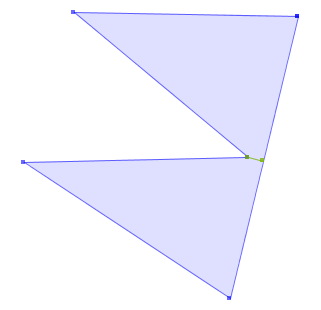org.locationtech.jts:jts-core 1.18.0
org.locationtech.jts.precision

## Class MinimumClearance

• ```public class MinimumClearance
extends Object```
Computes the Minimum Clearance of a `Geometry`.

The Minimum Clearance is a measure of what magnitude of perturbation of the vertices of a geometry can be tolerated before the geometry becomes topologically invalid. The smaller the Minimum Clearance distance, the less vertex perturbation the geometry can tolerate before becoming invalid.

The concept was introduced by Thompson and Van Oosterom [TV06], based on earlier work by Milenkovic [Mi88].

The Minimum Clearance of a geometry G is defined to be the value r such that "the movement of all points by a distance of r in any direction will guarantee to leave the geometry valid" [TV06]. An equivalent constructive definition [Mi88] is that r is the largest value such:

1. No two distinct vertices of G are closer than r
2. No vertex of G is closer than r to an edge of G of which the vertex is not an endpoint
The following image shows an example of the Minimum Clearance of a simple polygon.If G has only a single vertex (i.e. is a `Point`), the value of the minimum clearance is `Double.MAX_VALUE`.

If G is a `Puntal` or `Lineal` geometry, then in fact no amount of perturbation will render the geometry invalid. In this case a Minimum Clearance is still computed based on the vertex and segment distances according to the constructive definition.

It is possible for no Minimum Clearance to exist. For instance, a `MultiPoint` with all members identical has no Minimum Clearance (i.e. no amount of perturbation will cause the member points to become non-identical). Empty geometries also have no such distance. The lack of a meaningful MinimumClearance distance is detected and suitable values are returned by `getDistance()` and `getLine()`.

The computation of Minimum Clearance utilizes the `STRtree.nearestNeighbour(ItemDistance)` method to provide good performance even for large inputs.

An interesting note is that for the case of `MultiPoint`s, the computed Minimum Clearance line effectively determines the Nearest Neighbours in the collection.

### References

• [Mi88] Milenkovic, V. J., Verifiable implementations of geometric algorithms using finite precision arithmetic. in Artificial Intelligence, 377-401. 1988
• [TV06] Thompson, Rod and van Oosterom, Peter, Interchange of Spatial Data-Inhibiting Factors, Agile 2006, Visegrad, Hungary. 2006
Author:
Martin Davis
• ### Constructor Summary

Constructors
Constructor and Description
`MinimumClearance(Geometry geom)`
Creates an object to compute the Minimum Clearance for the given Geometry
• ### Method Summary

All Methods
Modifier and Type Method and Description
`double` `getDistance()`
Gets the Minimum Clearance distance.
`static double` `getDistance(Geometry g)`
Computes the Minimum Clearance distance for the given Geometry.
`LineString` `getLine()`
Gets a LineString containing two points which are at the Minimum Clearance distance.
`static Geometry` `getLine(Geometry g)`
Gets a LineString containing two points which are at the Minimum Clearance distance for the given Geometry.
• ### Methods inherited from class java.lang.Object

`equals, getClass, hashCode, notify, notifyAll, toString, wait, wait, wait`
• ### Constructor Detail

• #### MinimumClearance

`public MinimumClearance(Geometry geom)`
Creates an object to compute the Minimum Clearance for the given Geometry
Parameters:
`geom` - the input geometry
• ### Method Detail

• #### getDistance

`public static double getDistance(Geometry g)`
Computes the Minimum Clearance distance for the given Geometry.
Parameters:
`g` - the input geometry
Returns:
the Minimum Clearance distance
• #### getLine

`public static Geometry getLine(Geometry g)`
Gets a LineString containing two points which are at the Minimum Clearance distance for the given Geometry.
Parameters:
`g` - the input geometry
Returns:
the value of the minimum clearance distance or LINESTRING EMPTY if no Minimum Clearance distance exists
• #### getDistance

`public double getDistance()`
Gets the Minimum Clearance distance.

If no distance exists (e.g. in the case of two identical points) Double.MAX_VALUE is returned.

Returns:
the value of the minimum clearance distance or Double.MAX_VALUE if no Minimum Clearance distance exists
• #### getLine

`public LineString getLine()`
Gets a LineString containing two points which are at the Minimum Clearance distance.

If no distance could be found (e.g. in the case of two identical points) LINESTRING EMPTY is returned.

Returns:
the value of the minimum clearance distance or LINESTRING EMPTY if no Minimum Clearance distance exists